# Calculus 3 : Curl

## Example Questions

### Example Question #11 : Curl

Find the curl of the vector function: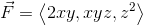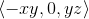#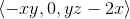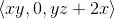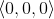#Explanation:

The curl of the function is given by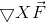First, we must write the determinant in order to take the cross product: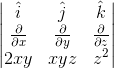where i, j, and k are the unit vectors corresponding to the x, y, and z direction respectively.

Next, we take the cross product. One can do this by multiplying across from the top left to the lower right, and continuing downward, and then subtracting the terms multiplied from top right to the bottom left: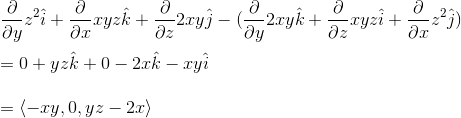The partial derivatives were found using the following rules: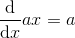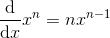### Example Question #12 : Curl

Find the curl of the vector function: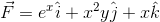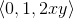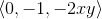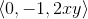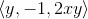Explanation:

The curl of the function is given byFirst, we must write the determinant in order to take the cross product: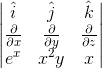where i, j, and k are the unit vectors corresponding to the x, y, and z direction respectively.

Next, we take the cross product. One can do this by multiplying across from the top left to the lower right, and continuing downward, and then subtracting the terms multiplied from top right to the bottom left: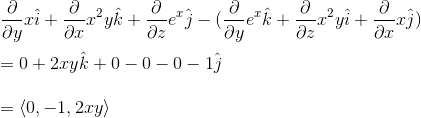The derivatives were found using the following rules:### Example Question #13 : Curl

Find the curl of the vector function: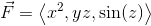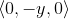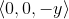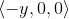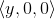Explanation:

The curl of a vector function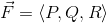is given by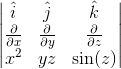where i, j, and k are the unit vectors corresponding to the x, y, and z direction respectively.

Next, we take the cross product. One can do this by multiplying across from the top left to the lower right, and continuing downward, and then subtracting the terms multiplied from top right to the bottom left. To find the given partial derivative of the function, we must treat the other variable(s) as constants: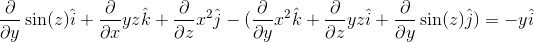The partial derivatives were found using the following rule:### Example Question #14 : Curl

Determine if the vector field is conservative or not, and why: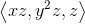The vector field is not conservative because the curl does not equal to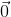.

The vector field is conservative because the curl is equal to.

The vector field is not conservative because the curl is equal to.

The vector field is conservative because the curl is not equal to.

The vector field is not conservative because the curl does not equal to.

Explanation:

The curl of the function is given by the cross product of the gradient and the vector function. If a vector function is conservative if the curl equals zero.

First, we can write the determinant in order to take the cross product of the two vectors: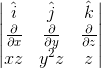where i, j, and k are the unit vectors corresponding to the x, y, and z direction respectively.

Next, we take the cross product. One can do this by multiplying across from the top left to the lower right, and continuing downward, and then subtracting the terms multiplied from top right to the bottom left: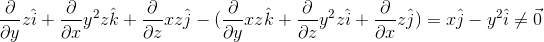### Example Question #15 : Curl

Find the curl of the following vector field, in vector form: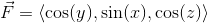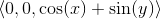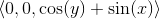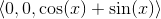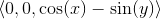Explanation:

The curl of the vector field is given by: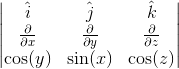where i, j, and k are the unit vectors corresponding to the x, y, and z direction respectively.

Next, we take the cross product. One can do this by multiplying across from the top left to the lower right, and continuing downward, and then subtracting the terms multiplied from top right to the bottom left. To find the given partial derivative of the function, we must treat the other variable(s) as constants.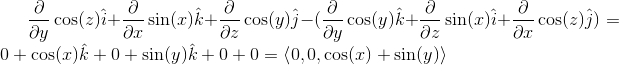### Example Question #14 : Curl

Find the curl of the following vector field, in vector form: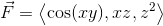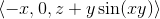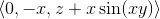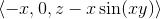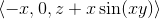Explanation:

The curl of the vector field is given by: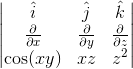where i, j, and k are the unit vectors corresponding to the x, y, and z direction respectively.

Next, we take the cross product. One can do this by multiplying across from the top left to the lower right, and continuing downward, and then subtracting the terms multiplied from top right to the bottom left. To find the given partial derivative of the function, we must treat the other variable(s) as constants.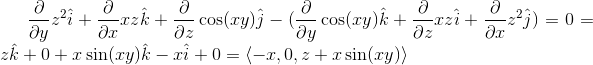### Example Question #15 : Curl

Find the curl of the following vector field: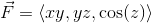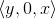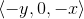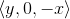Explanation:

The curl of the vector field is given by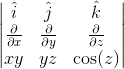Next, we take the cross product. One can do this by multiplying across from the top left to the lower right, and continuing downward, and then subtracting the terms multiplied from top right to the bottom left. To find the given partial derivative of the function, we must treat the other variable(s) as constants.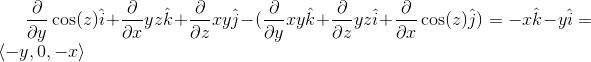### Example Question #16 : Curl

Determine the curl of the following vector field: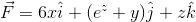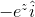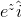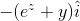Explanation:

The curl of the vector field is given by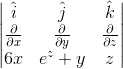where i, j, and k are the unit vectors corresponding to the x, y, and z direction respectively.

Next, we take the cross product. One can do this by multiplying across from the top left to the lower right, and continuing downward, and then subtracting the terms multiplied from top right to the bottom left. To find the given partial derivative of the function, we must treat the other variable(s) as constants.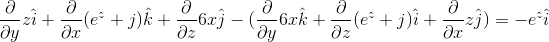### Example Question #17 : Curl

Determine the curl of the following vector field: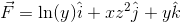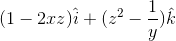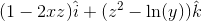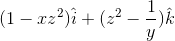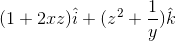Explanation:

The curl of the vector field is given by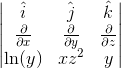where i, j, and k are the unit vectors corresponding to the x, y, and z direction respectively.

Next, we take the cross product. One can do this by multiplying across from the top left to the lower right, and continuing downward, and then subtracting the terms multiplied from top right to the bottom left. To find the given partial derivative of the function, we must treat the other variable(s) as constants.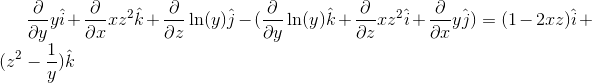### Example Question #18 : Curl

Find the curl of the following vector field: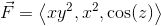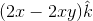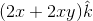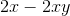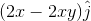Explanation:

The curl of the vector field is given by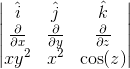where i, j, and k are the unit vectors corresponding to the x, y, and z direction respectively.

Next, we take the cross product. One can do this by multiplying across from the top left to the lower right, and continuing downward, and then subtracting the terms multiplied from top right to the bottom left. To find the given partial derivative of the function, we must treat the other variable(s) as constants.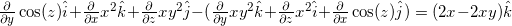### All Calculus 3 Resources